# 【数据结构与算法】查找算法（一）

## 一、概论

### 1.1 基本概念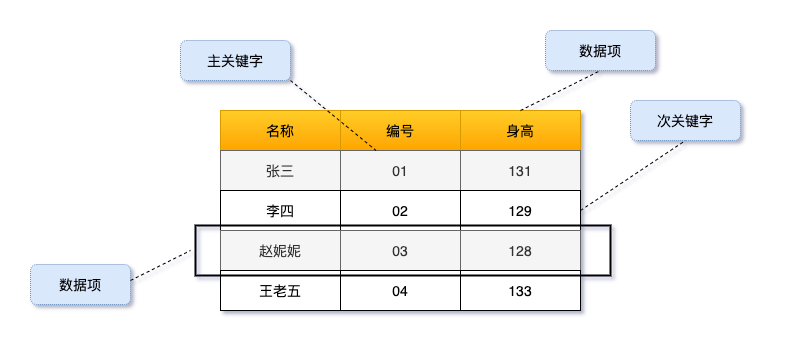### 1.2 分类

1. 查询某个“特定的”数据元素是否在查找表中
2. 检索某个“特点的“数据元素和各种属性

1. 查找时插入数据元素
2. 查找时删除数据元素

## 二、静态查找

### 2.1 顺序查找

1. 若某个记录的关键字和给定值相等，则查找成功，找到所查的记录；
2. 如果直到最后一个（或第一个）记录，其关键字和给定值比较都不等时，则表中没有所查的记录，查找不成功。

#### 2.3.1 顺序查找算法

int Sequential_Search(int *a, int n, int key)
{
int i;
for (i = 1; i<= n; i++) {
if (a[i] == key) {
return i;
}
}
return 0;
}

#### 2.3.2 顺序查找优化

int Sequential_Search(int *a, int n, int key)
{
int i;
// 1. 在0 位设置称为key，称为哨兵
a = key ;
i = n;

// 2. 从尾部开始倒序循环
while (a[i] != key) {
i--;
}
// 3. 返回>0 说明找到虚序号；=0 则 = key，表示查找失败
return i;
}

1. 在0 位设置称为key，称为哨兵
2. 循环倒置。从尾部开始倒序循环，好处是形成了一个完整循环（包括哨兵）
3. 查找结果二合一：返回>0 说明找到虚序号；=0 则 = key，表示查找失败

### 2.4 折半查找

• 在有序表中，去中间记录作为比较对象，若给定值与中间记录的关键字相等，则查找成功；
• 若给定值小于中间记录的关键字，则在中间记录作伴去继续查找；
• 若给定值大于中间记录的关键字中，则在中间记录的右半区继续查找。

// 折半查找
int Binary_Search(int *a, int n, int key)
{
int low, high, mid;
low = 1;
high = n;
while (low <= high) {
mid = (low + high) / 2;
if (key < a[mid]) {
high = mid - 1;
}else if(key > a[mid]){
low = mid + 1;
}else{
return mid;
}
}
return 0;
}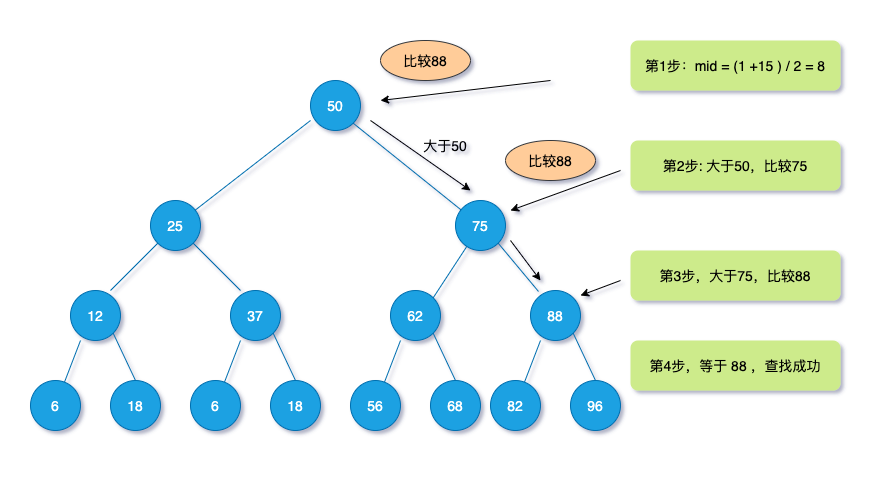### 2.3 插值查找

#### 2.3.1 折半改进

$$mid = \frac{low+high}{2} = low + \frac{1}{2} (high - low)$$

$$mid = low + \frac{ key - a [low]}{ a[high] - a[low]}(high - low)$$

#### 2.3.2 核心概念

$$\frac{key - a[low]}{a[high] - a[low]}$$

#### 2.3.2 算法实现

  // 插值查找
int Binary_Search(int *a, int n, int key)
{
int low, high, mid;
low = 1;
high = n;
while (low <= high) {
//  插值公示
mid = low + (high - low) * (key - a[low]) / (a[high] - a[low]));
if (key < a[mid]) {
high = mid - 1;
}else if(key > a[mid]){
low = mid + 1;
}else{
return mid;
}
}
return 0;
}

### 2.5 斐波那契查找

• 先定义一个斐波那契数列 F[n]

下标 0 1 2 3 4 5 6 7 8 9
F 0 1 1 2 3 5 8 13 21 34

它原理之前有学习过，实现原理是递归原理，代码实现如下：

      F = 0;
F = 1;
for (int i = 2; i < 100; i++) {
F[i] = F[i -1] + F[i - 2];
}
• 定义变量和下表

int low, high, mid, i, k;
low = 1, high = n;

其中 lowhigh分别为数组最低下标，默认 high为最大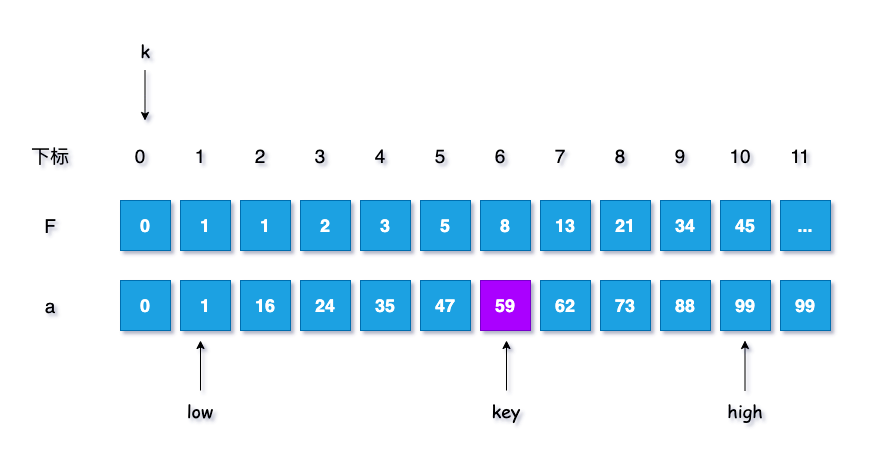• 计算 n 位于斐波那契数列的位置

while (n > F[k]) -1
k++;

由于上文给出的数组长度$n = 10$，得出 $F < n < F$， 所以此时 $k = 7$，在队列中如图示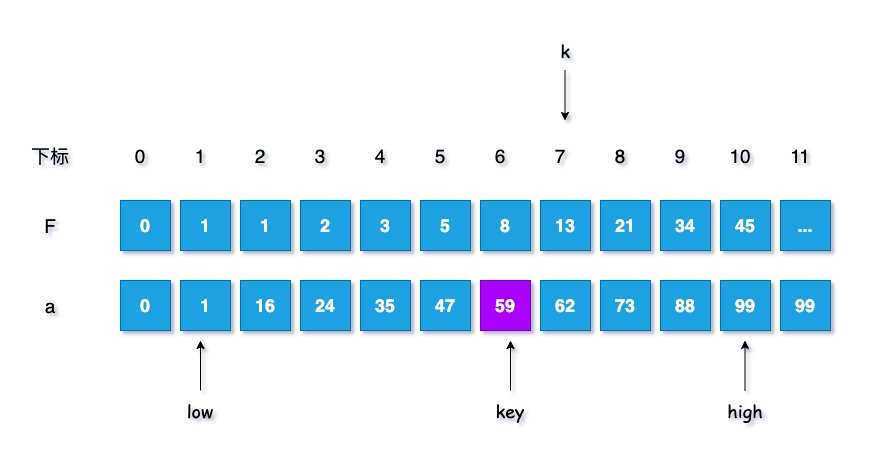• 将不满的数值补全，用a[n]为什么补齐？

    for (i = n; i < F[k] - 1; i++) {
a[i] = a[n];
}
• 开始查找：

1. 设定初始条件 $low <= high$，假设查找 n = 10，需要查找key = 59

2. 计算当前分割下标，$mid = low + F[k - 1] -1$， 此时$mid = 1 + F[7-1] -1 =8$

3. 判断，若 $key < a[mid]$ ，即如果查找记录小于当前分割记录

• 最高下标调整到分隔下标的 $mid -1$ 处

high = mid -1;
• 斐波那契数列下标减$1$位

k--;

这一步中 key 为59， a[mid] 及a 为73，所以$key<a[mid]$，需要进行调整， 如下图所示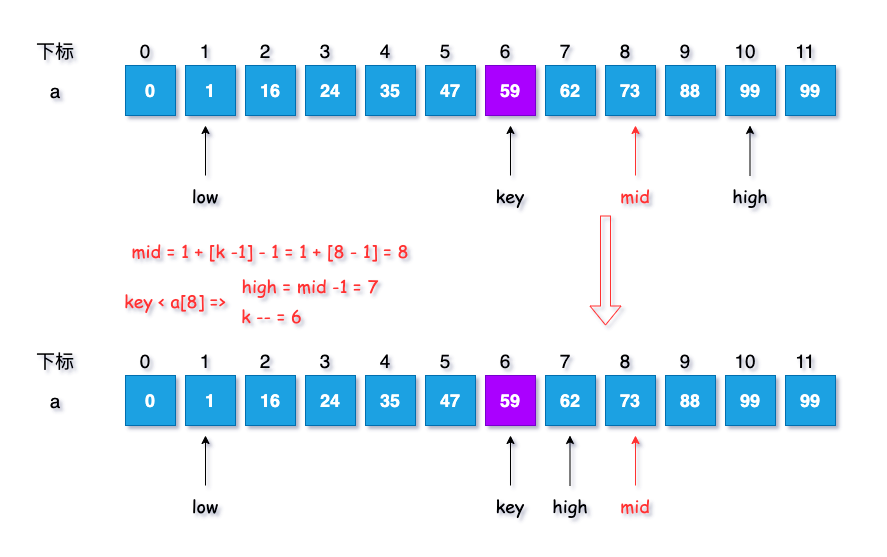此时各项参数结果为：$k = 6, low = 1, mid = 8, high = 7$

4. 继续循环查找。

1. 计算当前分隔的下标：$mid = F[6-1] - 1 = 5$ 。
2. 继续判断 key 与 a[mid]，此时 $a = 47 < key$，
3. 执行两个步骤：
• 最低下标调整到分割下标 $mid + 1$ 处

low = mid + 1;
• 斐波那契数列下标减2位

k = k - 2;

实现示意图如下：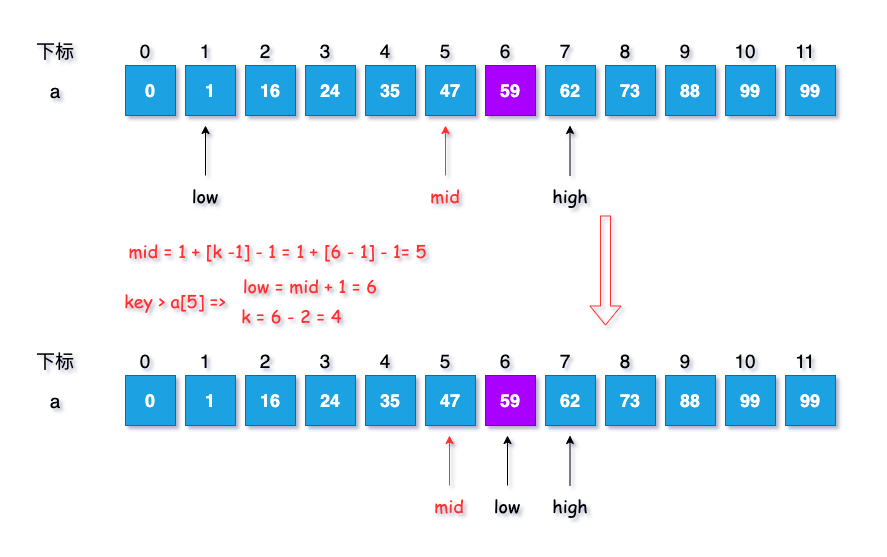此时各项参数结果为：$k = 4, low = 6, mid = 5, high = 7$

5. 再次循环。

1. 计算当前分隔的下标：$mid = 6 + F[4-1] -1 = 7$。
2. 继续判断 key 与 a[mid] 的大小，此时 $a = 62 > key$，因此执行第三步的策略。
3. 重复一下如下：
• 最高下标调整到分隔下标的 $mid -1$ 处

high = mid -1;
• 斐波那契数列下标减$1$位

k--

实现示意图如下：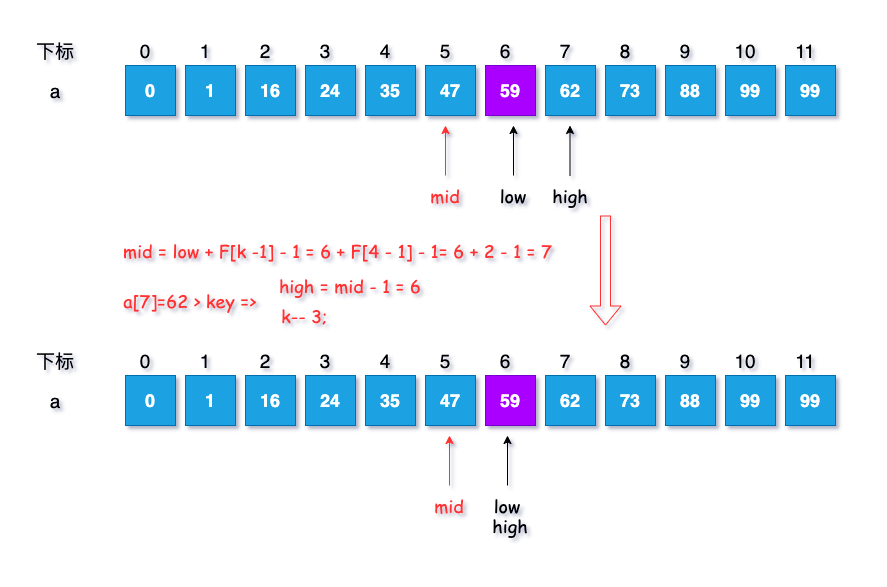此时各项参数结果为：$k = 3, low = 6, mid = 5, high = 6$

6. 再次循环。

1. 计算当前分隔的下标：$mid = 6 + F[3-1] =1 = 6$

2. 判断 key 与 a[mid] ，此时 a = 59 = key 。说明查找到，进行返回数组下标，返回值为 $6$

if(mid < n)
return mid;
else
return n;
• 回答为什么补齐$a[n]$

在循环查找的过程中，如果查找的$key=99$，

那么第一次查找时，

• $mid = 8$；
• $low = mid + 1 = 9$
• $k = k - 2 = 7 - 2 = 5$

第二次循环时，此时改变分隔mid 下标的值，$mid =low + F[k - 1] - 1 = 9 + 3 - 1 = 11$， 而此时a[mid] 为a ，数组a 中的11位时没有值，会导致下一步与 key 的比较失败，为了避免情况发生，对上文中将不满的数值补全，用 a[i] = a[n]

#### 2.2.2 算法核心

1. 当 $key = a[mid]$ 时，查找成功
2. 当 $key < a[mid]$ 时，新范围是第 low 个到第 mid -1 个，此时范围个数为 F[k - 1] - 1 个
3. 当 $key > a[mid]$ 时，新范围是第 m+1 个到第 high 个，此时范围个数为 F[k-2] -1 个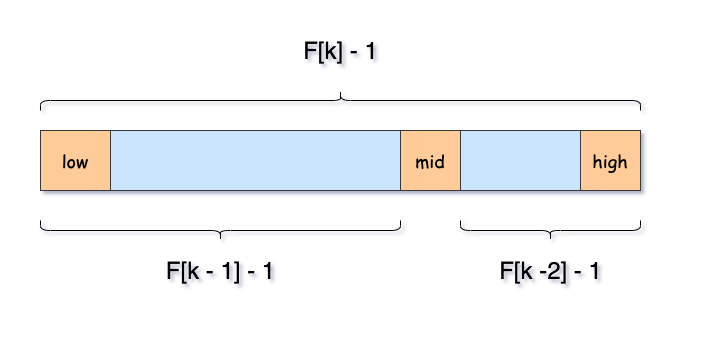#### 2.2.2 算法实现

int F;
int Fibonnaci_Search(int *a, int n, int key)
{
int low, high, mid, i, k;
low = 1;
high = n;
k = 0;
while (n > F[k] - 1) {
k++;
}
for (i = n; i < F[k] - 1; i++) {
a[i] = a[n];
}

while (low <= high) {
mid = low + F[k -1] -1;
if (key < a[mid]) {
high = mid -1;
k--;
}
else if (key > a[mid])
{
low = mid++;
k = k -2;
}
else
{
if (mid <= n)
return mid;
else
return n;
}
}
return 0;
}

### 2.6 查找效率

• 折半查找: $mid = (low + high) / 2$
• 插值查找，适合: $mid = low + \frac{key - a[low]}{a[high] - a[low]} * (high - low)$
• 斐波那契查找: $mid = low + F[k - 1] - 1$

• 折半查找：适合数据规模较小，执行效率高
• 插值查找：适合数据规模较大，比折半查找更高效，但规模较小，以数值递增波动较大时与折半查找区别不大；
• 斐波那契：相比插值查找，适合数据递增波动较大的场景。

## 三、动态查找

### 3.1 二叉排序树

#### 3.1.1 定义

• 若它的左子树不空，则右子树上所有结点的值均小于它的根结点的值
• 若它的右子树不空，则左子树上所有结点的值均大于它的根结点的值
• 它的左、右子树分别为二叉排序树

#### 3.1.2 查找

1. 二叉树的结构如下：

typedef struct BitNode{
int data;                                                 // 数据域
struct BitNode *lChild, *rChild; // 指针分别指向左、右子树
}BitNode, *BiTree;
2. 代码实现如下:

Status SearchBST(BiTree T, int key, BiTree f, BiTree *p)
{
if (!T) {
*p = f;
return FALSE;
}else if( key == T->data){
*p = T;
return SUCCESS;
}else if(key < T->data){
return SearchBST(T->lChild, key, T, p);
}else{
return SearchBST(T->rChild, key, T, p);
}
}
3. 分析查找流程:

1. 判断树的有效性
2. 指针 f 指向 T 结点的双亲，如果第一次，使用值为 NULL
3. 判断结点数值与 目标值元素结点的数据是否相等
1. 结点的值与目标值相等——指针 p 指向元素结点，返回成功
2. 如果目标值key小于元素结点数据，在左子树上，进行查找函数递归
3. 如果目标 key大于元素结点数据，在右子树上，进行查找函数递归
4. 示意图如下：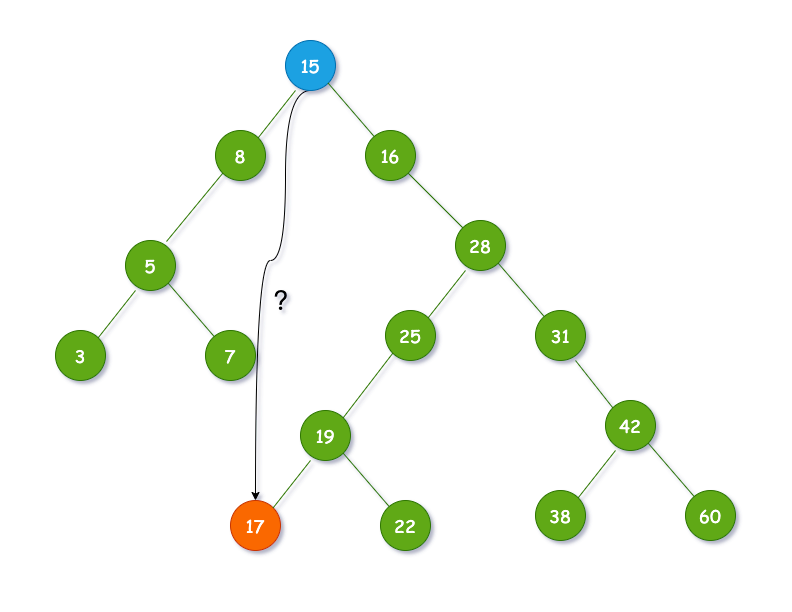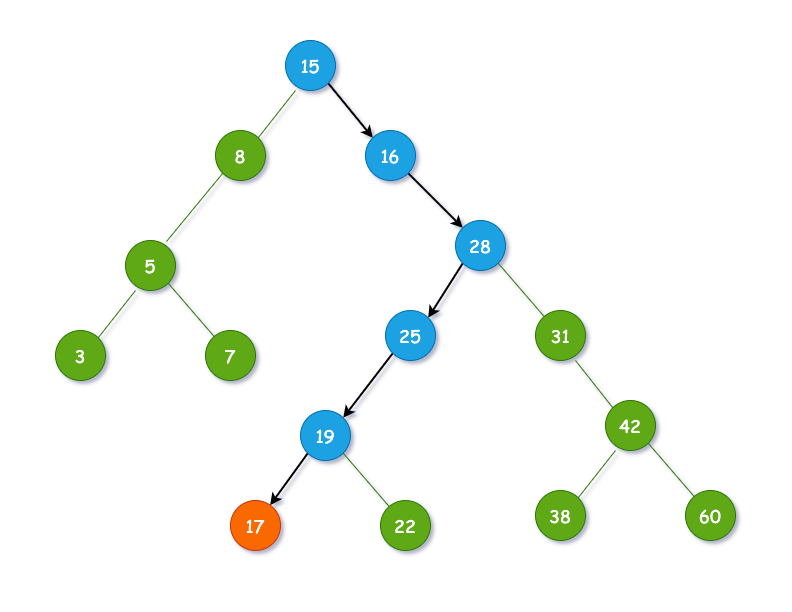#### 3.1.3 插入

• 先查找目标元素，如果存在，无需插入，返回
• 如果不存在，判断 目标值 key 与 指针p
• 如果大于，则 插入 s 为左孩子
• 如果小于，则插入 s 为右孩子

Status InsertBST(BiTree *T, int key)
{
BiTree p, s;
if (!SearchBST(*T, key, NULL, &p)) {
s = (BiTree)malloc(sizeof(BiTree));
s->data = key;
s->lChild = s->rChild = NULL;
if (!p) {
*T = s;
}else if (key < p->data){
p->lChild = s;
}else{
p->rChild = s;
}
return SUCCESS;
}else{
return FALSE;
}
}

#### 3.2.4 删除

• 叶子结点
• 仅有左/右子树的结点
• 左右子树都有结点的

• 当删除叶子结点：

无需操作，free 即可

• 当删除仅有左/右子树的结点时：

将它的原子树移动到它的位置，替代他即可

• 当删除结点左右子树都有结点时：

• 找到待删结点的前缀
• 重接q 的右子树
• 重接q 的左子树

Status DeleteBST(BiTree *p)
{
BiTree q,s;
// 右子树为空
if ((*p)->rChild == NULL) {
q = *p;
*p = (*p)->lChild;
free(q);

// 左子树为空
}else if ((*p)->lChild == NULL){
q = *p;
*p = (*p)->rChild;
free(q);

// 左右子树均不为空
}else{
q = *p;
s = (*p)->lChild;
while (s->rChild) {
q = s;
s = s->rChild;
}
(*p)->data = s->data;
if (q!= *p) {
q->rChild = s->lChild;
}else{
q->lChild = s->lChild;
}
free(s);
}
return SUCCESS;
}

• 找到目标结点 $8$，标记为 p
• 将p 赋值给临时变量 q，再将其左子树赋值给临时变量 s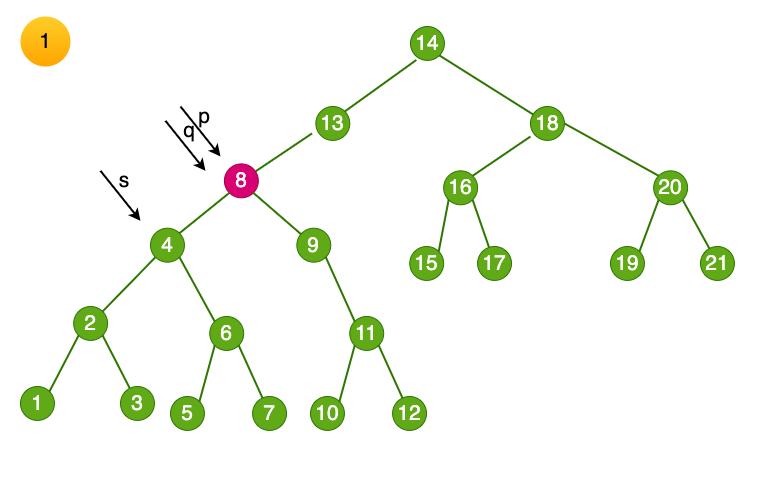• 循环找到左子树的右结点，知道右侧尽头，得到结点$7$，将临时变量s指向该结点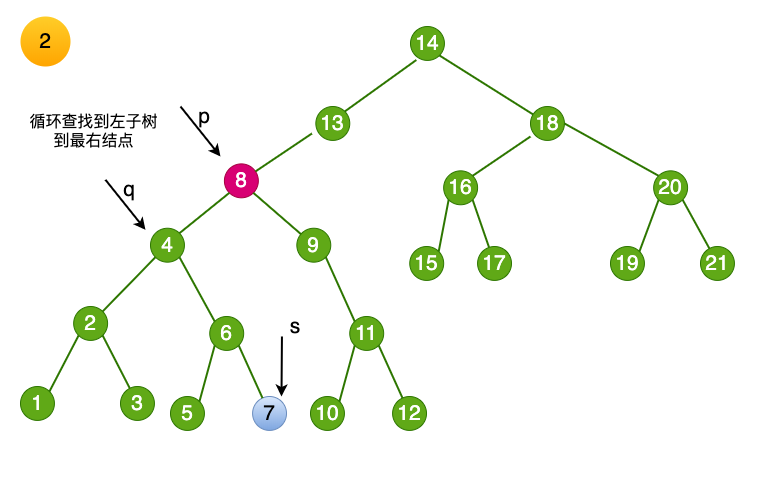• 将删除结点的数值赋值为 临时变量 s-> data，即 p->data = s->data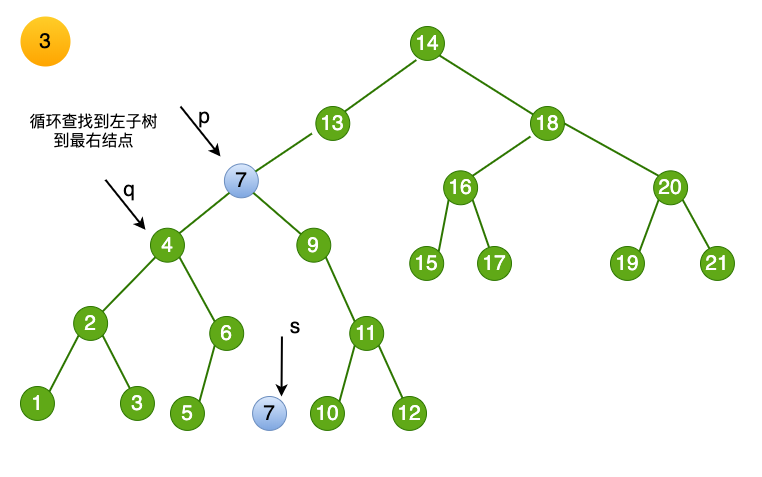• 最后一步，将目标结点7 进行释放，至此，删除完成。

• 这里的步骤和上文一样，赋值临时变量p，s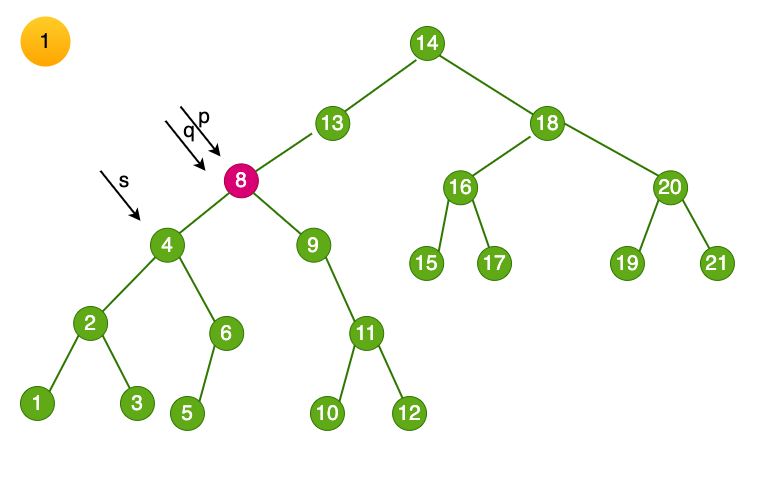• 找到目标结点6，将临时变量s 指向6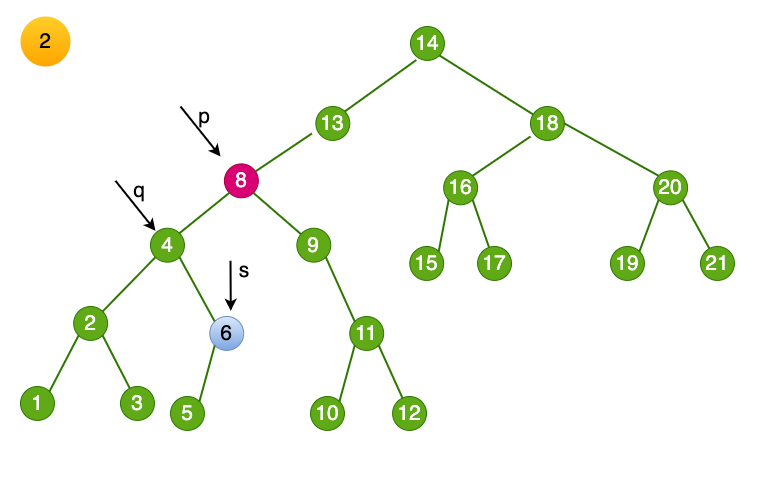• 将删除结点的数值赋值为 临时变量 s-> data，即 p->data = s->data。此时相当于结点6 被删除，它的子结点需要妥善处理。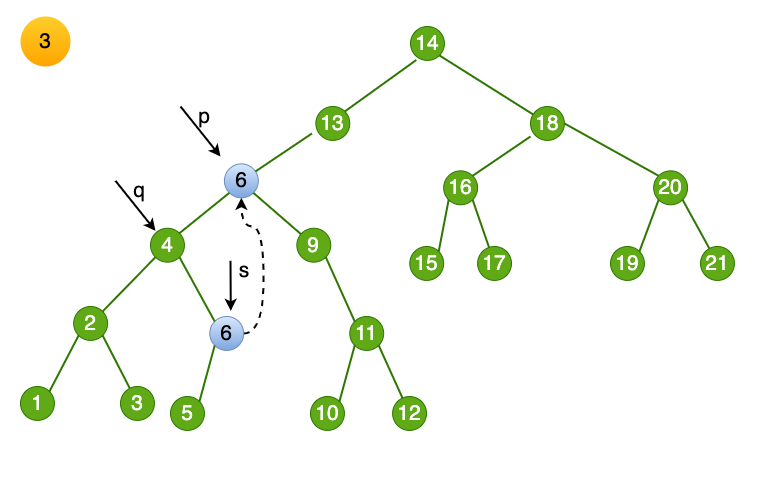• 此时如果 p 和 q 指向不相同，则将 6 的子树交给 它的父结点。

q->rChild = s->lChild。这样节点6 留下的左结点被链接进入树了。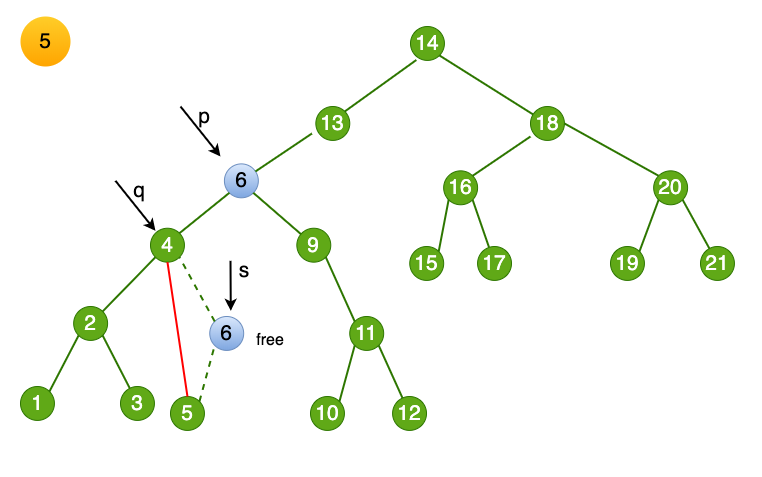• 最后一步，free 掉删除的目标结点#### 3.2.5 总结

上一篇【数据结构与算法】查找算法（二）平衡二叉树

2020-05-15 李佳【数据结构与算法10】拓扑排序

2020-05-13 李佳
目录